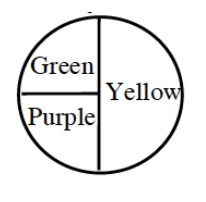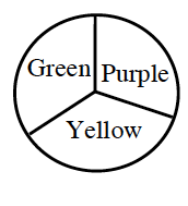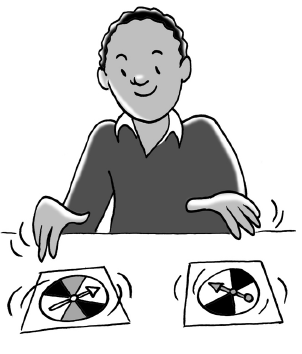### Home > CCG > Chapter 4 > Lesson 4.2.5 > Problem4-110

4-110.

When he was in first grade, Harvey played games with spinners. One game he especially liked had two spinners and several markers that you moved around a board. You were only allowed to move if your color came up on both spinners.1. Harvey always chose purple because that was his favorite color. What was the probability that Harvey could move his marker?

Review the Math Notes box in Lesson 4.2.3.

$\frac{1}{12}$

2. Is the event that Harvey wins a union or an intersection of events?

Refer to the Math Notes box in Lesson 4.2.4.

3. Was purple the best color choice? Explain.

Does purple have the greatest probability?

4. If both spinners are spun, what is the probability that no one gets to move because the two colors are not the same?

$\frac{1}{4} \cdot \frac{2}{3} = \frac{1}{6}$
$\frac{1}{6} + \frac{1}{6} + \frac{1}{3} = \frac{2}{3}$